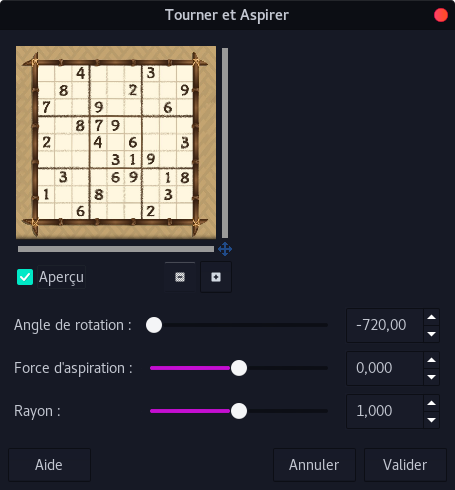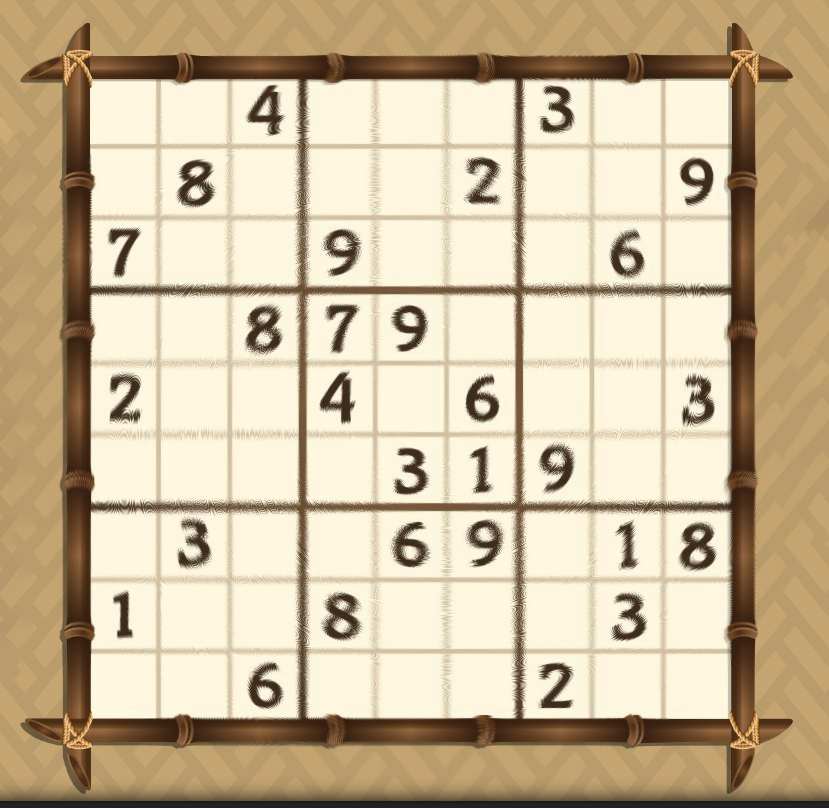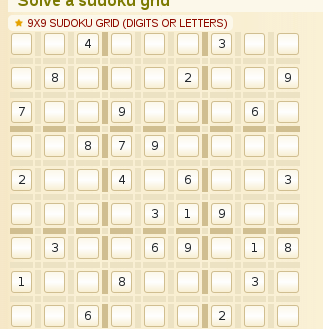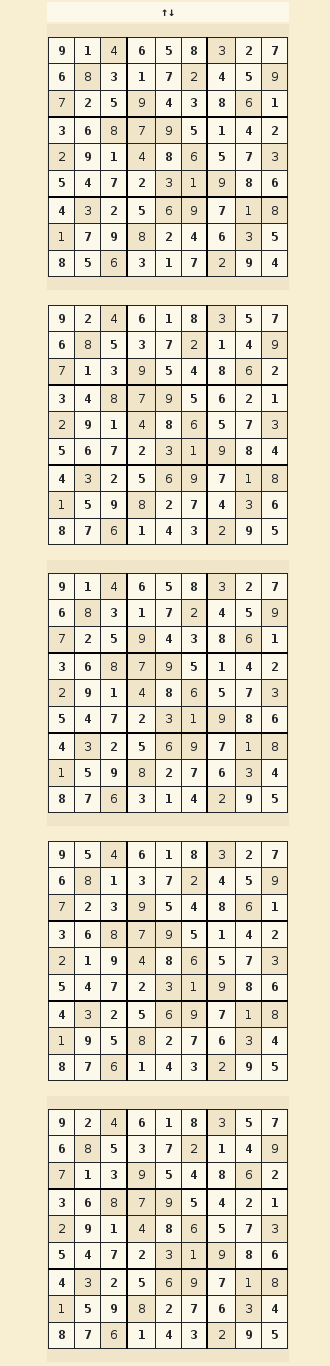# Sudo ku

Aperi'CTF 2019 - Steganography (250 pts).

# Aperi’CTF 2019 - Sudo ku

### Challenge details

Event Challenge Category Points Solves
Aperi’CTF 2019 Sudo ku Steganography 250 1

You wanna play a game ? Then follow the rules.

Challenge:
- YouLoose.jpg - md5sum: 5d4ccd32191d7dd6bb5823bab4e3b528

Resources: - Steganography using Sudoku Puzzle.pdf - md5sum: d9422e48a7931bc073ac1a35703176ea

### TL;DR

Use sudoku solver to get each sudoku solutions of YouLoose.jpg. Read the paper and implement the extract scheme. Test extract scheme on each solutions.

### Methodology

#### Whirlpinch

We got multiple files and an help (resources). One of the file is a sudoku which had a swirl effect. To remove it, open the file YouLoose.jpg with gimp or photoshop and apply the reversed effect. In gimp: Filters > Distorsion > Whirlpinch. Then set the rotation value to -720,00.Then export/save the file.#### Solve sudoku

If you attempt to solve the sudoku you’ll propably notice that there is multiple solutions for it. To get each solutions, we’ll use a sudoku solver. I found this sudoku solver which I ported to python3 (because I wanted a python3 solution).

I decided to write the following script to get each solutions:

#!/usr/bin/env python3
# -*- coding:utf-8 -*-

# http://infohost.nmt.edu/tcc/help/lang/python/examples/sudoku/
# (Ported to python3)
from sudosolver import *

s = "..4...3.." \
".8...2..9" \
"7..9...6." \
"..879...." \
"2..4.6..3" \
"....319.." \
".3..69.18" \
"1..8...3." \
"..6...2.."

SOLUTIONS = []

"""
SudokuSolver solutionObserver handler
Add each matrice solution to SOLUTIONS
"""
global SOLUTIONS
M = []
for rowx in range(9):
l = []
for colx in range(9):
cell = x.get(rowx, colx)
l.append(cell)
M.append(l)
SOLUTIONS.append(M)

slv.solve()

for num, sol in enumerate(SOLUTIONS):
print("[Solution n° "+str(num+1)+"]")
print(sol)

Output:

[Solution n° 1]
[[9, 1, 4, 6, 5, 8, 3, 2, 7],[6, 8, 3, 1, 7, 2, 4, 5, 9], [7, 2, 5, 9, 4, 3, 8, 6, 1], [3, 6, 8, 7, 9, 5, 1, 4, 2], [2, 9, 1, 4, 8, 6, 5, 7, 3], [5, 4, 7, 2, 3, 1, 9, 8, 6], [4, 3, 2, 5, 6, 9, 7, 1, 8], [1, 5, 9, 8, 2, 7, 6, 3, 4], [8, 7, 6, 3, 1, 4, 2, 9, 5]]
[Solution n° 2]
[[9, 1, 4, 6, 5, 8, 3, 2, 7], [6, 8, 3, 1, 7, 2, 4, 5, 9], [7, 2, 5, 9, 4, 3, 8, 6, 1], [3, 6, 8, 7, 9, 5, 1, 4, 2], [2, 9, 1, 4, 8, 6, 5, 7, 3], [5, 4, 7, 2, 3, 1, 9, 8, 6], [4, 3, 2, 5, 6, 9, 7, 1, 8], [1, 7, 9, 8, 2, 4, 6, 3, 5], [8, 5, 6, 3, 1, 7, 2, 9, 4]]
[Solution n° 3]
[[9, 2, 4, 6, 1, 8, 3, 5, 7], [6, 8, 5, 3, 7, 2, 1, 4, 9], [7, 1, 3, 9, 5, 4, 8, 6, 2], [3, 4, 8, 7, 9, 5, 6, 2, 1], [2, 9, 1, 4, 8, 6, 5, 7, 3], [5, 6, 7, 2, 3, 1, 9, 8, 4], [4, 3, 2, 5, 6, 9, 7, 1, 8], [1, 5, 9, 8, 2, 7, 4, 3, 6], [8, 7, 6, 1, 4, 3, 2, 9, 5]]
[Solution n° 4]
[[9, 2, 4, 6, 1, 8, 3, 5, 7], [6, 8, 5, 3, 7, 2, 1, 4, 9], [7, 1, 3, 9, 5, 4, 8, 6, 2], [3, 6, 8, 7, 9, 5, 4, 2, 1], [2, 9, 1, 4, 8, 6, 5, 7, 3], [5, 4, 7, 2, 3, 1, 9, 8, 6], [4, 3, 2, 5, 6, 9, 7, 1, 8], [1, 5, 9, 8, 2, 7, 6, 3, 4], [8, 7, 6, 1, 4, 3, 2, 9, 5]]
[Solution n° 5]
[[9, 5, 4, 6, 1, 8, 3, 2, 7], [6, 8, 1, 3, 7, 2, 4, 5, 9], [7, 2, 3, 9, 5, 4, 8, 6, 1], [3, 6, 8, 7, 9, 5, 1, 4, 2], [2, 1, 9, 4, 8, 6, 5, 7, 3], [5, 4, 7, 2, 3, 1, 9, 8, 6], [4, 3, 2, 5, 6, 9, 7, 1, 8], [1, 9, 5, 8, 2, 7, 6, 3, 4], [8, 7, 6, 1, 4, 3, 2, 9, 5]]

There is 5 possible solutions for this sudoku.

Note that https://www.dcode.fr also have a sudoku solver:#### PDF Paper

Since the challenge ask us to follow the rules, we’ll try to follow the paper “Steganography using Sudoku Puzzle” given in resources. This paper is about a steganography methode using solved sudoku and image.

We’ll take TheGame.png image as our payload to hide:In the process, the user has to compute a matrix named “reference matrix” which is the sudoku solved, duplicated to fill the size of the hidden image.

Here our image has the following size: 420*696. We have to duplicate our sudoku matrix in x and y to have a reference matrix with the size of 420*696.

Here is an example: if our solved sudoku had the size of 3*3 and contain only letters (usually 9*9 containing digits):

a b c
d e f
g h i

Then, with an image with the size of 11*11, we would have computed the following reference matrix:

a b c a b c a b c a b
d e f d e f d e f d e
g h i g h i g h i g h
a b c a b c a b c a b
d e f d e f d e f d e
g h i g h i g h i g h
a b c a b c a b c a b
d e f d e f d e f d e
g h i g h i g h i g h
a b c a b c a b c a b
d e f d e f d e f d e
#!/usr/bin/env python3
# -*- coding:utf-8 -*-

# http://infohost.nmt.edu/tcc/help/lang/python/examples/sudoku/
# (Ported to python3)
from PIL import Image
from sudosolver import *

s = "..4...3.." \
".8...2..9" \
"7..9...6." \
"..879...." \
"2..4.6..3" \
"....319.." \
".3..69.18" \
"1..8...3." \
"..6...2.."

SOLUTIONS = []

"""
SudokuSolver solutionObserver handler
Add each matrice solution to SOLUTIONS
"""
global SOLUTIONS
M = []
for rowx in range(9):
l = []
for colx in range(9):
cell = x.get(rowx, colx)
l.append(cell)
M.append(l)
SOLUTIONS.append(M)

def sudokuToRefMatrix(s, w, h):
"""
Convert sudoku "s" to a reference matrix with
size of "size"
"""
M = []
for i in range(h):
M.append((s[i % len(s)] * ((w//9)+1))[:w])
return M

slv.solve()

imgSrcName = "TheGame.png"
imgSrc = Image.open(imgSrcName)
w, h = imgSrc.size

for num, sol in enumerate(SOLUTIONS):
print("[Solution n° "+str(num+1)+"]")
refMatrix = sudokuToRefMatrix(sol,w,h)
print(len(refMatrix))
print(len(refMatrix))
[Solution n° 1]
696
420
[Solution n° 2]
696
420
[Solution n° 3]
696
420
[Solution n° 4]
696
420
[Solution n° 5]
696
420

We do not display each matrix but they are correct. Now here is the extract process: - The 9 first pixels contains the size n of the hidden data. Looking at the image, the 8 first pixels are black (0 value) and last is blue. Size is coded in binary mode (big endian) on 9 first pixels. - The next n pixels contains n positions in the reference matrix (x abscisse is coded on green channel and y on red channel). For a given position in the reference matrix we got a digit between 1 and 9.

Note: the paper had a mistake in it because they assume M(gi,gi+1) = M(R,G). However, gi is supposed to be Y abscisse and gi+1 X abscisse according to their Fig. 3 and wikipedia. This is in contradiction with one of their sentence: “Then R and G are chosen as X-axis and Y-axis”. In other word, a part of the text could be missunderstood due to a mistake. This can be solved by swapping R and G.

Once the n digits between 1 and 9 are extracted, we need to convert from base9 to int (base10) and then to ascii. If you do not understand the extract process, see Steganography using Sudoku Puzzle.pdf

Here is the final script which try to extract data for each reference matrix with coordinates defined by pixels values:

#!/usr/bin/env python3
# -*- coding:utf-8 -*-

import codecs
from PIL import Image

# http://infohost.nmt.edu/tcc/help/lang/python/examples/sudoku/
# (Ported to python3)
from sudosolver import *

def longToTxt(l):
hexa = hex(l)[2:].strip('L').encode("utf-8")
return codecs.decode(hexa, "hex_codec").decode("utf-8")

def base9ToInt(i):
""" Integer (base9) to Integer (base10) """
return int(i, 9)

"""
SudokuSolver solutionObserver handler
Add each matrice solution to SOLUTIONS
"""
global SOLUTIONS
M = []
for rowx in range(9):
l = []
for colx in range(9):
cell = x.get(rowx, colx)
l.append(cell)
M.append(l)
SOLUTIONS.append(M)

def subMatrix(M, n=1):
"""
Substract n to each elt in an 2D array
(substract 1 by default)
"""
return [[x-n for x in l] for l in M]

def sudokuToRefMatrix(s, w, h):
"""
Convert sudoku "s" to a reference matrix with
size of "size"
"""
M = []
for i in range(h):
M.append((s[i % len(s)] * ((w//9)+1))[:w])
return M

def tuplesToSize(t):
"""
Compute size from 9 firsts tuples of list t
"""
i = 0
nb = 0
for l in t[:9][::-1]:
for elt in l[::-1]:
nb += elt*(256**i)
i += 1
return nb

###############################################################################

s = "..4...3.." \
".8...2..9" \
"7..9...6." \
"..879...." \
"2..4.6..3" \
"....319.." \
".3..69.18" \
"1..8...3." \
"..6...2.."

imgSrcName = "TheGame.png"

c1 = 0  # Channel 1 is Red ==> 0
c2 = 1  # Channel 2 is Green ==> 1

SOLUTIONS = []  # List of solutions for the sudoku "s"

if __name__ == "__main__":

slv.solve()

imgSrc = Image.open(imgSrcName)
w, h = imgSrc.size
imgdata = list(imgSrc.getdata())  # List of (r,g,b)

# Size (9 px) Fig 6. General format of embedding data
sizeTuples = tuplesToSize(imgdata)

for num, sol in enumerate(SOLUTIONS):
print("[Solution n° "+str(num+1)+"]")
expected = subMatrix(sol)  # Fig 2. Sudoku solution after subtracting 1
refMatrix = sudokuToRefMatrix(expected, w, h)  # Fig 4. Ref. matrix
data = ""  # tmp flag
for i in range(sizeTuples):
px = imgdata[i+9]  # +9 due to size hidding
data += str(refMatrix[px[c1]][px[c2]])  # extract data

try:  # If data extracted from refMatrix is printable then print it
print(longToTxt(base9ToInt(data)))
except:
continue

Output:

[Solution n° 1]
[Solution n° 2]
Bravo ! Vous pouvez valider le challenge avec le flag suivant: APRK{*5UD0KU_M4ST3R*}.
Congratz ! You can validate the challenge with the following flag: APRK{*5UD0KU_M4ST3R*}.

[Solution n° 3]
[Solution n° 4]
[Solution n° 5]

#### Flag

APRK{*5UD0KU_M4ST3R*}

You can find generic scripts to hide / extract data: - Hide data using sudoku : sudoku.py - md5sum : 83e3a26ee072575a10fcfc7c6c80b737 - Extract data using sudoku : sudoku_solve.py - md5sum : fcce0c40ec4e5e4bfc6f5690f08a83ca - Sudoku solver : sudosolver.py - md5sum : f9d6e578a69b29cdec483086df89478f

Zeecka